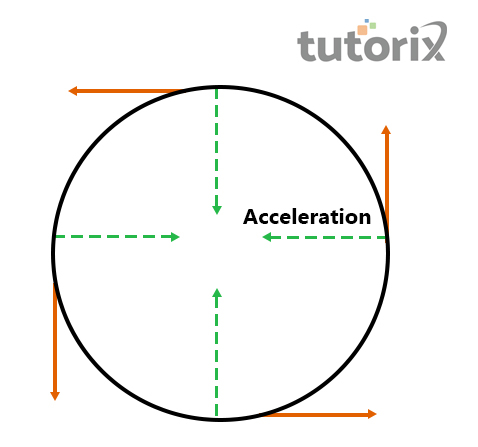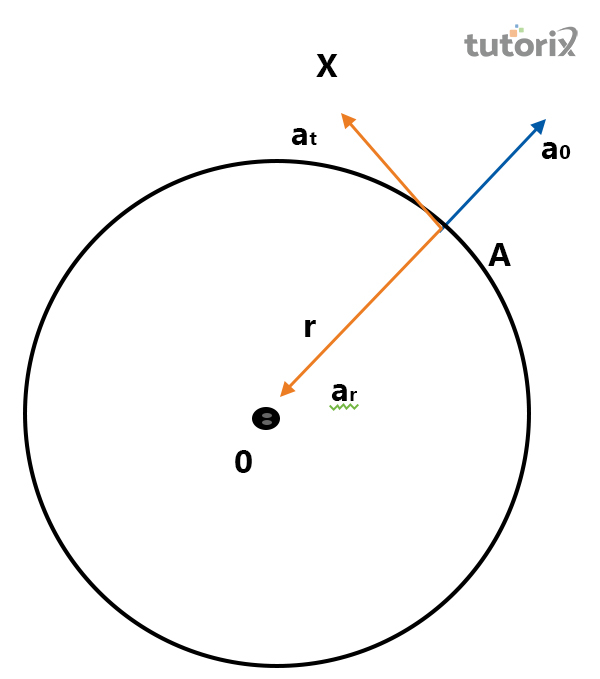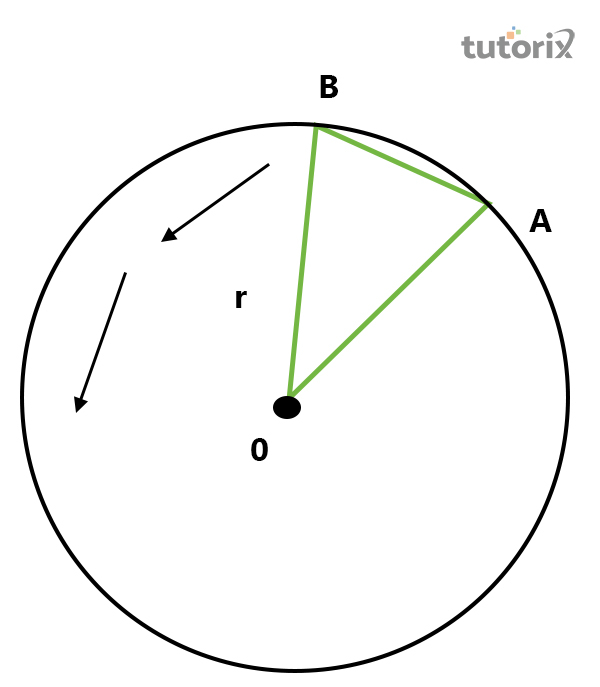## Introduction

Radial acceleration is commonly seen when the external force is applied to an object and according to the Second law of Newton acceleration none on the velocity of a particular object in respect of time. It includes the vector quantity that refers to both magnitudes as well as the direction of the force.

The measurement unit of radial acceleration is $\mathrm{ms^{-2}}$. Simply, the radial acceleration refers to the particular proportion of the velocity and the total time taken by an object. As per the two types of motion of an object, the radial acceleration is also different

The motion of an object is linear as well as circular motion. Therefore, the acceleration of an object is also two different types as per the motions are radial acceleration and tangential acceleration.

The symbol of Radial acceleration is a is it constantly changed with the angular velocity of the object along with the direction of movement of the object. The proportion of the movement, velocity as well as the direction of movement of an object is considered as the radial acceleration. It can be defined as the changing velocity in refers to a particular time.

The radial acceleration is considered as the acceleration of a particular object which is directed towards the centre of that object. This type of acceleration mainly takes place in a circular motion while the movement of the objects is concerned with the object’s radius.

The measurement unit of the radial acceleration of an object is two different types radians per second squared and meters per second squared.Figure 1: Circular motion

The radians per second squared is denoted by $\mathrm{\omega s^{-2}}$ and $\mathrm{ms^{-2}}$. The unit of angular displacement of an object is radian, and the unit of angular velocity is $\mathrm{radian/sec}$. $\mathrm{radian/sec2}$ is the unit of angular acceleration.

• Angular acceleration is classified into two different categories like radial as well as tangential acceleration.

• Radial acceleration is mainly referred to as the acceleration of the object-directed into the centre of the object.

• Radial acceleration can be measured by the unit of $\mathrm{\omega s^{-2}}$ in the case of radians are represented in per second square.

• Radial acceleration is also commonly popular as the centripetal acceleration

• The tangential acceleration is referred to as the principal component of angular acceleration tangential.

• Radial acceleration can be always represented as the instantaneous velocity so it is commonly known as general or common acceleration.

• It is called centripetal acceleration because this type of acceleration is mostly directed into the instantaneous centre of the trajectory’s curvature.

• This type of motion was never defined for the circular motion only rather than the different types of motion.

The radial acceleration depends on some components that affect the rate of acceleration. Angular displacement is a type of component that can be defined as the angular comparison of the preliminary as well as the ultimate position of a particular object. The symbol of this component is 𝜃 and its formula is $\mathrm{\theta = s/r}$.

The unit of angular displacement is the radian. Angular velocity is another type of component that can be defined as the changing rate of the angular displacement in terms of the particular time. The symbol of this component ⍵ and its formula is $\mathrm{\omega = d \theta /dt}$ (Brouwer et al. 2021). The unit of angular displacement is the $\mathrm{radian/sec}$. Angular acceleration is another type of component that can be defined as the changing rate of the angular velocity in terms of a particular time (Stone & Courteau, 2019).

The symbol of this component $\mathrm{\alpha}$ and its formula is $\mathrm{\alpha = \frac{d \omega}{dt}}$. The unit of angular displacement is the $\mathrm{radian/sec2}$.

### The formula of Radial AccelerationFigure 2: Schematic diagram of an object of rotational motion

Object A is connected with another object with a string and when it is rotated in terms of a fixed point that is O, it looks like a circle radius. Therefore, in this case, the a0 acceleration is created with the redial direction.Figure 3: Radial acceleration of two objects

In the case of two different objects the radial acceleration can be measured with the formula $\mathrm{AB\:=\:v\:\times\:dt}$ where A and B are the two objects and it is dependent on v which refers to the speed and dt strands for different of time (Pradyumna & Desai, 2021).

In case of these object is very close then the formula changes to $\mathrm{v\:+\:dv\:≈\:dv\:\times\:AB/OA}$ which can aslso be presented as $\mathrm{dv/v\:=\:v^2/r}$. In the case of tangential acceleration the formula is $\mathrm{at\:=\:v^2-v1/t}$ where, at refers to the tangential components, t refers to the time period and $\mathrm{v^2}$ or $\mathrm{v^3}$ denotes the consequence of velocity of two different objects gets from the circular motion.

## Conclusion

The magnitude of the radial acceleration at any type of instant is $\mathrm{v^2/r}$ where v stands for the speed of the object and r is the curvature’s radius at any type of instant. In terms of circular motion, $\mathrm{r}$ refers to the radius and the radial acceleration direction and the curvature’s radius is the same. In terms of UCM (uniform circular motion), the tangential acceleration is defined as zero all time because the speed of the object is the same. In the case of any kind of rectangular motion, the radial acceleration is zero all time as the curvature's radius is in a straight line refers to as infinite.

### FAQs

Q1. What are acceleration as well as deceleration?

The acceleration, as well as deceleration, is the changing velocity of the object. The rate of these two particles depends on time.

Q2. What are the types of angular motion?

Angular motion is two different types of radial acceleration as well as tangential acceleration. Booth the type depends on the speed and time.

Q.3 What is referred to as angular velocity?

The time rate of angular displacement is called angular velocity. The formula for it is $\mathrm{(\omega) = (\delta \theta /\delta t)}$.

Q4. What are the types of acceleration?

Linear acceleration and circular acceleration are the two types of acceleration. The object of an object that moves along a curved trajectory comprises non-zero radial acceleration.

Updated on: 13-Oct-2022

2K+ Views